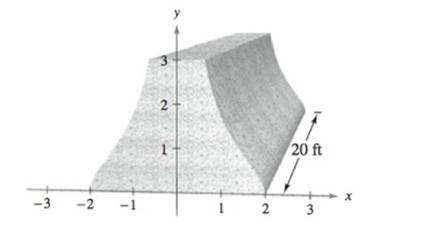Chapter 8.6, Problem 72E

Chapter
Section
Textbook Problem

# Building Design The cross section of a precast concrete beam for a building is bounded by the graphs of the equations x = 2 1 + y 2 ,   x = − 2 1 + y 2 ,   y = 0 ,   and   y = 3 where x and y are measured in feet. The length of the beam is 20 feet (see figure).(a) Find the volume V and the weight W of the beam. Assume the concrete weighs 148 pounds per cubic foot.(b) Find the centroid of a cross section of the beam.

(a)

To determine

To calculate: The volume V and the weight W of the beam where x=21+y2,x=21+y2,y=0 and y=3

Explanation

Given:

The cross section of a concrete beam is bounded by graphs of x=21+y2,x=21+y2,y=0 and y=3 as show below.

The length of the beam is 20 feet, and concrete has density of 148 pounds per cubic foot.

Formula used:

The volume is found by the formula:

V=0aA(y)dy

The integration formula:

1u2+a2du=ln|u+u2+a2|+C

Calculation:

Thevolume is,

V=20

(b)

To determine

To calculate: The centroid of the cross section of the beam, where cross section is bounded by x=21+y2,x=21+y2,y=0 and y=3

### Still sussing out bartleby?

Check out a sample textbook solution.

See a sample solution

#### The Solution to Your Study Problems

Bartleby provides explanations to thousands of textbook problems written by our experts, many with advanced degrees!

Get Started

#### In Exercises 7-10, solve for x or y. 7+y2=3

Calculus: An Applied Approach (MindTap Course List)

#### (13)0

Applied Calculus for the Managerial, Life, and Social Sciences: A Brief Approach

#### In problems 13-16, Use notation to indicate which set is a subset of the other. 15.

Mathematical Applications for the Management, Life, and Social Sciences